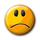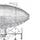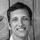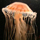## General Question# How do I calculate what size air conditioner (in BTUs) I need according to my apartment's square footage?

Asked by GD_Kimble (2277) July 17th, 2007

There's supposed to be some formula for this, but I can't locate it online anywhere.

Observing members: 0Composing members: 0Will also depend on insulation, windows, and locale (BTU requirement in San Francisco, for instance, is substantially lower than Phoenix, Arizona).

However, I can't seem to find the book with the general formula's in it... sorry!

bpeoples (2551)“Great Answer” (1) Flag as…For a rough estimate, multiply the volume of the room in cubic feet by 2.5 and then add 1,000 for every window and 400 for every occupant.

For example, if a room measures 12' × 20' × 10' with two windows and five occupants, the formula would be 2,400 cubic feet × 2.5 = 6,000. Add 2,000 for the windows and 2,000 for the occupants and you would need about a 10,000 BTU air conditioner.

In addition to the factors mentioned by bpeoples, you should take into account the number and direction of exposed walls, but this formula should be a good starting point.

sferik (6106)“Great Answer” (1) Flag as…Sferik, how did you come by that formula?

rosedog (225)“Great Answer” (0) Flag as…I started with a formula from Ace Hardware.

This formula only takes into account the square footage of the room. I decided that the height of the room ought to be factored in, so I added a dimension and then divided the multiplier by 10 (assuming that they assumed an average room to have a ten-foot high ceiling).

Again, this is just a rough estimate. Ace Hardware may have rigged the formula to sell more-powerful-than-necessary (and therefore more expensive) air conditioners, but I did compare this formula with formulae on other websites and found it to be about right.

The Association of Home Appliance Manufacturers offers a more complete formula that includes sun exposure, insulation levels, and a variety of other variables. There is another, simpler calculator from This Old House.

If I use the later to calculate the necessary cooling capacity for the same hypothetical 12' × 20' × 10' room, with a southern exposure, no kitchen, insulation, and direct sunlight, it results in the same 10,000 BTU estimate.

sferik (6106)“Great Answer” (1) Flag as…Response moderated

or